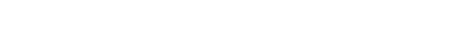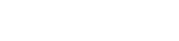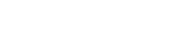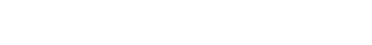10 Most Common AP Calculus BC Mistakes (and How to Avoid Them!)

So if you’re planning to take the AP Calculus BC exam, then you probably know your stuff. Nevertheless, you’ll want to watch out for these ten common AP Calculus BC exam mistakes.Can you get past all of the obstacles on the AP Calculus BC exam?

Top 10 AP Calculus BC Exam Mistakes

In my experience, students who sign up for the BC exam are generally tops in their class. They are probably going to pursue a STEM major at a prestigious college or university.

With that being said, though, certain errors can creep up on the best of us.

So here are some of the most common Calculus BC exam mistakes.

1. Not Showing Enough Work

You should be aware that the AP Calculus BC exam has two sections, Multiple Choice (MC) and Free Response (FR).

You must show your work on every FR question. Otherwise, the graders will not give you full credit even if your answer is correct.

If you use a major calculator function (like numerical integration), write down the integral itself, the result, and a sentence explaining that you used your calculator to compute it.

In addition, you might want to check out these helpful tips to conquering AP Calculus Free Response questions

When using a calculator, be sure to keep all of the digits in your work until the very last step. And even then, don’t round too much.

As a silly example, suppose the question asks for the value of 22/7 – π.

Both 22/7 and π are roughly equal to 3.14, so is the answer 0?

Let’s take a closer look: 22/7 ≈ 3.1428571, while π ≈ 3.1415926.

Therefore 22/7 – π is about 0.0012645. That’s a tiny number, but not equal to 0.

3. Sequences are Not Series

A sequence is just a list of numbers.

A series is the sum of a list of numbers.

They are not the same concepts.

However, some of the same terminology is used with sequences as with series, and this is where confusion may arise.

We say a sequence (an) converges if the limit exists as n → ∞.

But a series converges only if its partial sums approach a definite value.

For example, the harmonic sequence, 1, 1/2, 1/3, 1/4, 1/5, …, converges to 0. But the harmonic series, 1 + 1/2 + 1/3 + 1/4 + 1/5 + …., diverges.

4. Powers Versus Derivatives

Keep in mind that the nth derivative of a function is often written with a superscript (n) if n > 3. Some students mistake these higher-order derivatives for powers of the function.5. Derivatives, Velocity, and Speed

You should know this in your sleep: The derivative of position is equal to velocity.

Sometimes, however, students forget how to find the speed of an object.

• For a one-variable function, speed is equal to the absolute value of velocity.
• For a vector function, speed is equal to the magnitude of the velocity vector.

6. Integration By Parts Issues

It’s essential to have memorized the Integration by Parts (IBP) formula:Not only that, but you must have experience using it. Don’t forget that the minus sign in the middle applies to the entire integral, ∫ v du.

This is especially important if you have to do multiple IBP. Make sure to use parentheses to group all of the terms of ∫ v du together once you have worked out that integral.

7. Messing Up the Logic in Comparison Test

Proving that a series converges or diverges often relies on subtle mathematical reasoning. The most common errors that I have come across involve the direction of the inequalities in comparison test.

Suppose we are trying to prove whether a series Σ an converges or diverges by comparing its terms to another (simpler) series, Σ bn.

Here’s a handy chart.

If Σ bn converges...If Σ bn diverges...
...and if anbnΣ an converges.No conclusion
...and if anbnNo conclusionΣ an diverges.

8. Average Value Does Not Mean Arithmetic Mean

There are two related concepts in calculus that are called “average something,” and neither one should be done by adding up a bunch of items and dividing.

Average rate of change (or average velocity) is the change in position divided by the change in time. In other words,The average value of a function on a given interval is found by taking a certain definite integral.9. Using Euler’s Method Unnecessarily

Euler’s Method does show up on the BC test. However, I don’t recommend using it unless the question asks you to do so.

The best way to handle an initial value problem is to solve the differential equation explicitly.
Euler’s Method only gives approximate solutions.

10. Not Checking the Endpoints of the Interval of Convergence

When you study power series, you learn how to get the radius (R) and center of convergence (a). Then the interval of convergence must be one of the following intervals:

• [aR, a + R]
• (aR, a + R]
• [aR, a + R)
• (aR, a + R)

But how do you choose among these?

The answer is that you must plug in each endpoint, x = aR and x = a + R, into the power series. Then, you have to analyze both of these numerical series for convergence or divergence. When the corresponding series converges, then that endpoint must be included in the interval of convergence.

Summary

Of course, these are not the only errors that students make, but they are some of the most common Calculus BC exam mistakes.

For a list of common mistakes on the AB exam, check out: 10 Most Common AP Calculus AB Exam Mistakes (and How to Avoid Them!)

Improve your SAT or ACT score, guaranteed. Start your 1 Week Free Trial of Magoosh SAT Prep or your 1 Week Free Trial of Magoosh ACT Prep today!Author

•Shaun earned his Ph. D. in mathematics from The Ohio State University in 2008 (Go Bucks!!). He received his BA in Mathematics with a minor in computer science from Oberlin College in 2002. In addition, Shaun earned a B. Mus. from the Oberlin Conservatory in the same year, with a major in music composition. Shaun still loves music -- almost as much as math! -- and he (thinks he) can play piano, guitar, and bass. Shaun has taught and tutored students in mathematics for about a decade, and hopes his experience can help you to succeed!